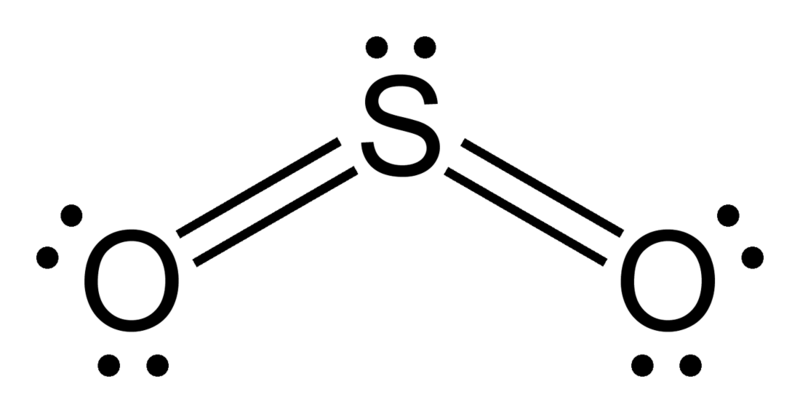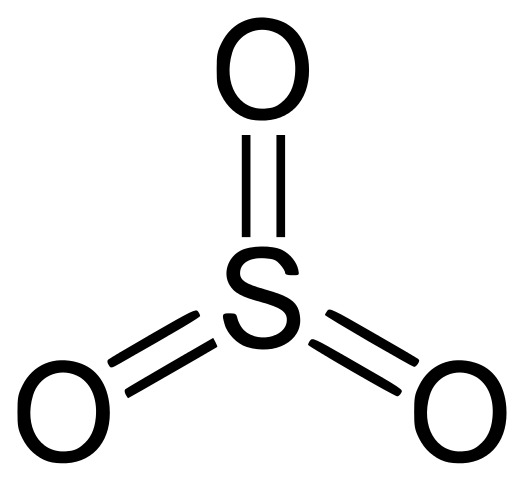# Difference Between SO2 and SO3

## Main Difference – SO2 vs SO3

SO2 and SO3 are inorganic chemical compounds formed by the combination of sulfur atoms and oxygen atoms. SO2 stands for sulfur dioxide, and SO3 stands for sulfur trioxide. These are gaseous compounds. They have different chemical and physical properties. These compounds are called oxides of sulfur since they are formed from the reaction between sulfur and O2 molecules. The main difference between SO2 and SO3 is that SO2 has two oxygen atoms bonded to a sulfur atom whereas SO3 has three oxygen atoms bonded to a sulfur atom.

### Key Areas Covered

1. What is SO2
– Definition, Chemical Structure and Properties, Oxidation State
2. What is SO3
– Definition, Chemical Structure and Properties, Sulfuric Acid Production
3. What is the Difference Between SO2 and SO3
– Comparison of Key Differences

Key Terms: Acid Rain, Lone Electron Pair, Oxygen, Oxidation State, Sulfur, Sulfur Dioxide, Sulfuric Acid, Sulfur Trioxide## What is SO2

SO2 stands for sulfur dioxide. Sulfur dioxide is a gaseous compound composed of sulfur and oxygen atoms. The chemical formula of sulfur dioxide is SO2. Therefore, it is composed of a sulfur atom bonded to two oxygen atoms through covalent bonds. One oxygen atom can form a double bond with the sulfur atom. Hence, the sulfur atom is the central atom of the compound. Since sulfur element has 6 electrons in its outermost orbital after forming two double bonds with the oxygen atoms, there are 2 more electrons remaining; these can act as a lone electron pair. This determines the geometry of the SO2 molecule as angular geometry. SO2 is polar due to its geometry (angular) and the presence of a lone electron pair.Figure 1: Chemical Structure of SO2

Sulfur dioxide is considered as a toxic gas. Hence, if there is SO2 in the atmosphere, it will be an indication of air pollution. This gas has a very irritating smell. The molecular mass of sulfur dioxide is 64 g/mol. It is a colorless gas at room temperature. The melting point is about -71oC whereas the boiling point is -10oC.

The oxidation state of sulfur in sulfur dioxide is +4. Therefore, sulfur dioxide can also be produced by the reduction of compounds composed of sulfur atoms that are in a higher oxidation state. One such example is the reaction between copper and sulfuric acid. Here, sulfur in sulfuric acid is in the oxidation state of +6. Therefore, it can be reduced to +4 oxidation state of sulfur dioxide.

Sulfur dioxide can be used in the production of sulfuric acid which has a number of applications in the industrial scale and the laboratory scale. Sulfur dioxide is also a good reducing agent. Since the oxidation state of sulfur is +4 in sulfur dioxide, it can easily be oxidized to +6 oxidation state, which allows another compound to be reduced.

## What is SO3

SO3 stands for sulfur trioxide. Sulfur trioxide is a solid compound composed of one sulfur atom bonded to three oxygen atoms. The chemical formula of sulfur dioxide is SO3. Each oxygen has formed a double bond with the sulfur atom. The sulfur atom is in the center of the molecule. Since sulfur has 6 electrons in its outermost orbital, after forming three double bonds with the oxygen atoms, there are no more electrons remaining on sulfur atom. This determines the geometry of the SO3 molecule as trigonal planar geometry. SO3 is non-polar due to its geometry (trigonal planar) and the absence of a lone electron pair.Figure 2: Chemical Structure of SO3

The molecular mass of sulfur trioxide is 80.057 g/mol. The melting point of SO3 is about 16.9 °C whereas the boiling point is 45oC. At room temperature and pressure, sulfur trioxide is a white crystalline solid compound which will fume in the air. It has a pungent odor. The oxidation state of sulfur in sulfur trioxide is +6.

In its gaseous form, sulfur trioxide is an air pollutant and is a major component in acid rains. However, sulfur trioxide is very important in the production of sulfuric acid in industrial scale. This is because sulfur trioxide is the anhydride form of sulfuric acid.

SO3(l)    +    H2O(l)        →      H2SO4(l)

The above reaction is very rapid and exothermic. Therefore, control methods should be used when using sulfur trioxide for industrial sulfuric acid production. In addition, sulfur trioxide is an essential reagent in Sulfonation process.

## Difference Between SO2 and SO3

### Definition

SO2: SO2 stands for sulfur dioxide.

SO3: SO3 stands for sulfur trioxide.

### Nature

SO2: SO2 is a gaseous compound composed of sulfur and oxygen atoms.

SO3: SO3 is a solid compound composed of one sulfur atom bonded to three oxygen atoms.

### Molar Mass

SO2: The molar mass of SO2 is 64 g/mol.

SO3: The molar mass of SO3 is 80.057 g/mol.

### Melting Point and Boiling Point

SO2: The melting point of SO2 is about -71°C whereas the boiling point is -10°C.

SO3: The melting point of SO3 is about 16.9 °C whereas the boiling point is 45°C.

### Oxidation State

SO2: The oxidation state of sulfur in SO2 is +4.

SO3: The oxidation state of sulfur in SO3 is +6.

### Oxidation

SO2: SO2 can be further oxidized.

SO3: SO3 cannot be further oxidized.

### Polarity

SO2: SO2 is polar due to its geometry (angular) and the presence of a lone electron pair.

SO3: SO3 is non-polar due to its geometry (trigonal planar) and the absence of a lone electron pair.

### Conclusion

SO2 and SO3 are inorganic compounds that are named as sulfur oxides. SO2 is a gaseous compound at room temperature. SO3 is a solid (crystalline) compound at room temperature. The main difference between SO2 and SO3 is that SO2 has two oxygen atoms bonded to a sulfur atom whereas SO3 has three oxygen atoms bonded to a sulfur atom.

##### Reference:

1. “Sulfur dioxide.” Wikipedia, Wikimedia Foundation, 3 Jan. 2018, Available here.
2. “SULFUR TRIOXIDE.” National Center for Biotechnology Information. PubChem Compound Database, U.S. National Library of Medicine, Available here.
3. “SO3 Molecular Geometry, Lewis Structure, and Polarity Explained.” Geometry of Molecules, 21 July 2017, Available here.

##### Image Courtesy:

1. “Sulfur-dioxide-ve-B-2D” By Ben Mills – Own work (Public Domain) via Commons Wikimedia
2. “SO3 Sulfur trioxide” By Yikrazuul – Own work (Public Domain) via Commons Wikimedia